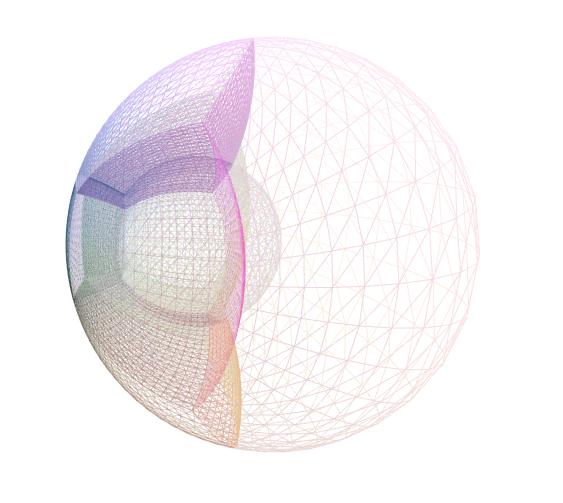SpECTRE  v2023.05.16
domain::CoordinateMaps::SpecialMobius Class Reference

Redistributes gridpoints within the unit sphere. More...

#include <SpecialMobius.hpp>

## Public Member Functions

SpecialMobius (double mu)

SpecialMobius (SpecialMobius &&)=default

SpecialMobius (const SpecialMobius &)=default

SpecialMobiusoperator= (const SpecialMobius &)=default

SpecialMobiusoperator= (SpecialMobius &&)=default

template<typename T >
std::array< tt::remove_cvref_wrap_t< T >, 3 > operator() (const std::array< T, 3 > &source_coords) const

std::optional< std::array< double, 3 > > inverse (const std::array< double, 3 > &target_coords) const
Returns std::nullopt for target_coords outside the unit sphere. The inverse function is only callable with doubles because the inverse might fail if called for a point out of range, and it is unclear what should happen if the inverse were to succeed for some points in a DataVector but fail for other points.

template<typename T >
tnsr::Ij< tt::remove_cvref_wrap_t< T >, 3, Frame::NoFramejacobian (const std::array< T, 3 > &source_coords) const

template<typename T >
tnsr::Ij< tt::remove_cvref_wrap_t< T >, 3, Frame::NoFrameinv_jacobian (const std::array< T, 3 > &source_coords) const

void pup (PUP::er &p)

bool is_identity () const

## Static Public Attributes

static constexpr size_t dim = 3

## Friends

bool operator== (const SpecialMobius &lhs, const SpecialMobius &rhs)

## Detailed Description

Redistributes gridpoints within the unit sphere.A sphere with a mu of 0.25.

### Details

A special case of the conformal Mobius transformation that maps the unit ball to itself. This map depends on a single parameter, mu $$= \mu$$, which is the x-coordinate of the preimage of the origin under this map. This map has the fixed points $$x=1$$ and $$x=-1$$. The map is singular for $$\mu=1$$ but we have found that this map is accurate up to 12 decimal places for values of $$\mu$$ up to 0.96.

We define the auxiliary variables

$r := \sqrt{x^2 + y^2 +z^2}$

and

$\lambda := \frac{1}{1 - 2 x \mu + \mu^2 r^2}$

The map corresponding to this transformation in cartesian coordinates is then given by:

$\vec{x}'(x,y,z) = \lambda\begin{bmatrix} x(1+\mu^2) - \mu(1+r^2)\\ y(1-\mu^2)\\ z(1-\mu^2)\\ \end{bmatrix}$

The inverse map is the same as the forward map with $$\mu$$ replaced by $$-\mu$$.

This map is intended to be used only inside the unit sphere. A point inside the unit sphere maps to another point inside the unit sphere. The map can have undesirable behavior at certain points outside the unit sphere: The map is singular at $$(x,y,z) = (1/\mu, 0, 0)$$ (which is outside the unit sphere since $$|\mu| < 1$$). Moreover, a point on the $$x$$-axis arbitrarily close to the singularity maps to an arbitrarily large value on the $$\pm x$$-axis, where the sign depends on which side of the singularity the point is on.

A general Mobius transformation is a function on the complex plane, and takes the form $$f(z) = \frac{az+b}{cz+d}$$, where $$z, a, b, c, d \in \mathbb{C}$$, and $$ad-bc\neq 0$$.

The special case used in this map is the function $$f(z) = \frac{z - \mu}{1 - z\mu}$$. This has the desired properties:

• The unit disk in the complex plane is mapped to itself.
• The x-axis is mapped to itself.
• $$f(\mu) = 0$$.

The three-dimensional version of this map is obtained by rotating the disk in the plane about the x-axis.

This map is useful for performing transformations along the x-axis that preserve the unit disk. A concrete example of this is in the BBH domain, where two BBHs with a center-of-mass at x= $$\mu$$ can be shifted such that the new center of mass is now located at x=0. Additionally, the spherical shape of the outer wave-zone is preserved and, as a mobius map, the spherical coordinate shapes of the black holes is also preserved.

The documentation for this class was generated from the following file:
• src/Domain/CoordinateMaps/SpecialMobius.hpp